# solve

Solve heat transfer or structural analysis problem

## Syntax

``structuralStaticResults = solve(structuralStatic)``
``structuralModalResults = solve(structuralModal,'FrequencyRange',[omega1,omega2])``
``structuralTransientResults = solve(structuralTransient,tlist)``
``structuralFrequencyResponseResults = solve(structuralFrequencyResponse,flist)``
``structuralTransientResults = solve(structuralTransient,tlist,'ModalResults',modalresults)``
``structuralFrequencyResponseResults = solve(structuralFrequencyResponse,flist,'ModalResults',modalresults)``
``thermalSteadyStateResults = solve(thermalSteadyState)``
``thermalTransientResults = solve(thermalTransient,tlist)``

## Description

example

````structuralStaticResults = solve(structuralStatic)` returns the solution to the static structural analysis model represented in `structuralStatic`.```

example

````structuralModalResults = solve(structuralModal,'FrequencyRange',[omega1,omega2])` returns the solution to the modal analysis model for all modes in the frequency range `[omega1,omega2]`. Define `omega1` as slightly smaller than the lowest expected frequency and `omega2` as slightly larger than the highest expected frequency. For example, if the lowest expected frequency is zero, then use a small negative value for `omega1`.```

example

````structuralTransientResults = solve(structuralTransient,tlist)` returns the solution to the transient structural dynamics model represented in `structuralTransient`.```

example

````structuralFrequencyResponseResults = solve(structuralFrequencyResponse,flist)` returns the solution to the frequency response model represented in `structuralFrequencyResponse`.```

example

````structuralTransientResults = solve(structuralTransient,tlist,'ModalResults',modalresults)` and `structuralFrequencyResponseResults = solve(structuralFrequencyResponse,flist,'ModalResults',modalresults)` solve a transient and a frequency response structural model, respectively, by using the modal superposition method to speed up computations. First, perform modal analysis to compute natural frequencies and mode shapes in a particular frequency range. Then, use this syntax to invoke the modal superposition method. The accuracy of the results depends on the modes in the modal analysis results.```

example

````thermalSteadyStateResults = solve(thermalSteadyState)` returns the solution to the steady-state thermal model represented in `thermalSteadyState`.```

example

````thermalTransientResults = solve(thermalTransient,tlist)` returns the solution to the transient thermal model represented in `thermalTransient` at the times `tlist`.```

## Examples

collapse all

Solve a 3-D steady-state thermal problem.

Create a thermal model for this problem.

`thermalmodel = createpde('thermal');`

Import and plot the block geometry.

```importGeometry(thermalmodel,'Block.stl'); pdegplot(thermalmodel,'FaceLabel','on','FaceAlpha',0.5) axis equal```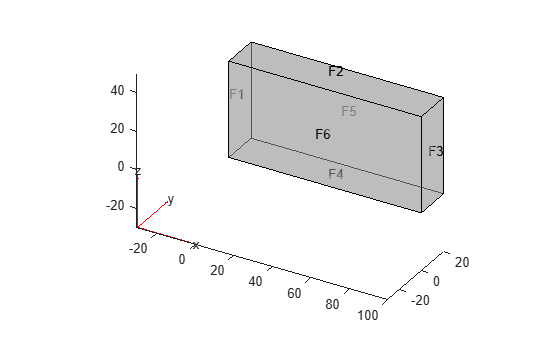Assign material properties.

`thermalProperties(thermalmodel,'ThermalConductivity',80);`

Apply a constant temperature of 100 °C to the left side of the block (face 1) and a constant temperature of 300 °C to the right side of the block (face 3). All other faces are insulated by default.

```thermalBC(thermalmodel,'Face',1,'Temperature',100); thermalBC(thermalmodel,'Face',3,'Temperature',300);```

Mesh the geometry and solve the problem.

```generateMesh(thermalmodel); thermalresults = solve(thermalmodel)```
```thermalresults = SteadyStateThermalResults with properties: Temperature: [12691x1 double] XGradients: [12691x1 double] YGradients: [12691x1 double] ZGradients: [12691x1 double] Mesh: [1x1 FEMesh] ```

The solver finds the temperatures and temperature gradients at the nodal locations. To access these values, use `thermalresults.Temperature`, `thermalresults.XGradients`, and so on. For example, plot temperatures at the nodal locations.

`pdeplot3D(thermalmodel,'ColorMapData',thermalresults.Temperature)`Solve a 2-D transient thermal problem.

Create a transient thermal model for this problem.

`thermalmodel = createpde('thermal','transient');`

Create the geometry and include it in the model.

```SQ1 = [3; 4; 0; 3; 3; 0; 0; 0; 3; 3]; D1 = [2; 4; 0.5; 1.5; 2.5; 1.5; 1.5; 0.5; 1.5; 2.5]; gd = [SQ1 D1]; sf = 'SQ1+D1'; ns = char('SQ1','D1'); ns = ns'; dl = decsg(gd,sf,ns); geometryFromEdges(thermalmodel,dl); pdegplot(thermalmodel,'EdgeLabels','on','FaceLabels','on') xlim([-1.5 4.5]) ylim([-0.5 3.5]) axis equal```For the square region, assign these thermal properties:

• Thermal conductivity is $10\text{\hspace{0.17em}}\mathrm{W}/\left(\mathrm{m}{\cdot }^{\circ }\mathrm{C}\right)$

• Mass density is $2\text{\hspace{0.17em}}\mathrm{kg}/{\mathrm{m}}^{3}$

• Specific heat is $0.1\text{\hspace{0.17em}}\mathrm{J}/\left({\mathrm{kg}\cdot }^{\circ }\mathrm{C}\right)$

```thermalProperties(thermalmodel,'ThermalConductivity',10, ... 'MassDensity',2, ... 'SpecificHeat',0.1, ... 'Face',1);```

For the diamond region, assign these thermal properties:

• Thermal conductivity is $2\text{\hspace{0.17em}}\mathrm{W}/\left(\mathrm{m}{\cdot }^{\circ }\mathrm{C}\right)$

• Mass density is $1\text{\hspace{0.17em}}\mathrm{kg}/{\mathrm{m}}^{3}$

• Specific heat is $0.1\text{\hspace{0.17em}}\mathrm{J}/\left({\mathrm{kg}\cdot }^{\circ }\mathrm{C}\right)$

```thermalProperties(thermalmodel,'ThermalConductivity',2, ... 'MassDensity',1, ... 'SpecificHeat',0.1, ... 'Face',2);```

Assume that the diamond-shaped region is a heat source with a density of $4\text{\hspace{0.17em}}\mathrm{W}/{\mathrm{m}}^{2}$.

`internalHeatSource(thermalmodel,4,'Face',2);`

Apply a constant temperature of $0{\phantom{\rule{0.16666666666666666em}{0ex}}}^{\circ }C$ to the sides of the square plate.

`thermalBC(thermalmodel,'Temperature',0,'Edge',[1 2 7 8]);`

Set the initial temperature to 0 °C.

`thermalIC(thermalmodel,0);`

Generate the mesh.

`generateMesh(thermalmodel);`

The dynamics for this problem are very fast. The temperature reaches a steady state in about 0.1 seconds. To capture the interesting part of the dynamics, set the solution time to `logspace(-2,-1,10)`. This command returns 10 logarithmically spaced solution times between 0.01 and 0.1.

`tlist = logspace(-2,-1,10);`

Solve the equation.

`thermalresults = solve(thermalmodel,tlist)`
```thermalresults = TransientThermalResults with properties: Temperature: [1481x10 double] SolutionTimes: [1x10 double] XGradients: [1481x10 double] YGradients: [1481x10 double] ZGradients: [] Mesh: [1x1 FEMesh] ```

Plot the solution with isothermal lines by using a contour plot.

```T = thermalresults.Temperature; pdeplot(thermalmodel,'XYData',T(:,10),'Contour','on','ColorMap','hot')```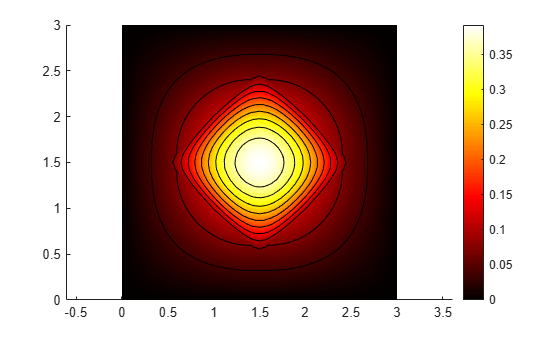Solve a static structural model representing a bimetallic cable under tension.

Create a static structural model for solving a solid (3-D) problem.

`structuralmodel = createpde('structural','static-solid');`

Create the geometry and include it in the model. Plot the geometry.

```gm = multicylinder([0.01,0.015],0.05); structuralmodel.Geometry = gm; pdegplot(structuralmodel,'FaceLabels','on','CellLabels','on','FaceAlpha',0.5)```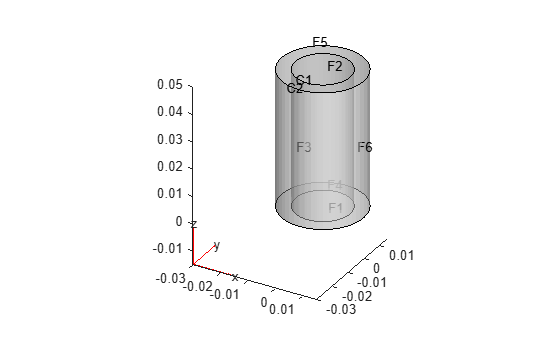Specify the Young's modulus and Poisson's ratio for each metal.

```structuralProperties(structuralmodel,'Cell',1,'YoungsModulus',110E9, ... 'PoissonsRatio',0.28); structuralProperties(structuralmodel,'Cell',2,'YoungsModulus',210E9, ... 'PoissonsRatio',0.3);```

Specify that faces 1 and 4 are fixed boundaries.

`structuralBC(structuralmodel,'Face',[1,4],'Constraint','fixed');`

Specify the surface traction for faces 2 and 5.

`structuralBoundaryLoad(structuralmodel,'Face',[2,5],'SurfaceTraction',[0;0;100]);`

Generate a mesh and solve the problem.

```generateMesh(structuralmodel); structuralresults = solve(structuralmodel)```
```structuralresults = StaticStructuralResults with properties: Displacement: [1x1 FEStruct] Strain: [1x1 FEStruct] Stress: [1x1 FEStruct] VonMisesStress: [22281x1 double] Mesh: [1x1 FEMesh] ```

The solver finds the values of displacement, stress, strain, and von Mises stress at the nodal locations. To access these values, use `structuralresults.Displacement`, `structuralresults.Stress`, and so on. The displacement, stress, and strain values at the nodal locations are returned as `FEStruct` objects with the properties representing their components.

`structuralresults.Displacement`
```ans = FEStruct with properties: ux: [22281x1 double] uy: [22281x1 double] uz: [22281x1 double] Magnitude: [22281x1 double] ```
`structuralresults.Stress`
```ans = FEStruct with properties: sxx: [22281x1 double] syy: [22281x1 double] szz: [22281x1 double] syz: [22281x1 double] sxz: [22281x1 double] sxy: [22281x1 double] ```
`structuralresults.Strain`
```ans = FEStruct with properties: exx: [22281x1 double] eyy: [22281x1 double] ezz: [22281x1 double] eyz: [22281x1 double] exz: [22281x1 double] exy: [22281x1 double] ```

Plot the deformed shape with the z-component of normal stress.

```pdeplot3D(structuralmodel,'ColorMapData',structuralresults.Stress.szz, ... 'Deformation',structuralresults.Displacement)```Solve for the transient response of a thin 3-D plate under a harmonic load at the center.

Create a transient dynamic model for a 3-D problem.

`structuralmodel = createpde('structural','transient-solid');`

Create the geometry and include it in the model. Plot the geometry.

```gm = multicuboid([5,0.05],[5,0.05],0.01); structuralmodel.Geometry=gm; pdegplot(structuralmodel,'FaceLabels','on','FaceAlpha',0.5)```Zoom in to see the face labels on the small plate at the center.

```figure pdegplot(structuralmodel,'FaceLabels','on','FaceAlpha',0.25) axis([-0.2 0.2 -0.2 0.2 -0.1 0.1])```Specify the Young's modulus, Poisson's ratio, and mass density of the material.

```structuralProperties(structuralmodel,'YoungsModulus',210E9,... 'PoissonsRatio',0.3,... 'MassDensity',7800);```

Specify that all faces on the periphery of the thin 3-D plate are fixed boundaries.

`structuralBC(structuralmodel,'Constraint','fixed','Face',5:8);`

Apply a sinusoidal pressure load on the small face at the center of the plate.

`structuralBoundaryLoad(structuralmodel,'Face',12,'Pressure',5E7,'Frequency',25);`

Generate a mesh with linear elements.

`generateMesh(structuralmodel,'GeometricOrder','linear','Hmax',0.2);`

Specify zero initial displacement and velocity.

`structuralIC(structuralmodel,'Displacement',[0;0;0],'Velocity',[0;0;0]);`

Solve the model.

```tlist = linspace(0,1,300); structuralresults = solve(structuralmodel,tlist)```
```structuralresults = TransientStructuralResults with properties: Displacement: [1x1 FEStruct] Velocity: [1x1 FEStruct] Acceleration: [1x1 FEStruct] SolutionTimes: [1x300 double] Mesh: [1x1 FEMesh] ```

The solver finds the values of the displacement, velocity, and acceleration at the nodal locations. To access these values, use `structuralresults.Displacement`, `structuralresults.Velocity`, and so on. The displacement, velocity, and acceleration values are returned as `FEStruct` objects with the properties representing their components.

`structuralresults.Displacement`
```ans = FEStruct with properties: ux: [1873x300 double] uy: [1873x300 double] uz: [1873x300 double] Magnitude: [1873x300 double] ```
`structuralresults.Velocity`
```ans = FEStruct with properties: vx: [1873x300 double] vy: [1873x300 double] vz: [1873x300 double] Magnitude: [1873x300 double] ```
`structuralresults.Acceleration`
```ans = FEStruct with properties: ax: [1873x300 double] ay: [1873x300 double] az: [1873x300 double] Magnitude: [1873x300 double] ```

Find the fundamental (lowest) mode of a 2-D cantilevered beam, assuming prevalence of the plane-stress condition.

Specify the following geometric and structural properties of the beam, along with a unit plane-stress thickness.

```length = 5; height = 0.1; E = 3E7; nu = 0.3; rho = 0.3/386;```

Create a model plane-stress model, assign a geometry, and generate a mesh.

```structuralmodel = createpde('structural','modal-planestress'); gdm = [3;4;0;length;length;0;0;0;height;height]; g = decsg(gdm,'S1',('S1')'); geometryFromEdges(structuralmodel,g);```

Define a maximum element size (five elements through the beam thickness).

```hmax = height/5; msh=generateMesh(structuralmodel,'Hmax',hmax);```

Specify the structural properties and boundary constraints.

```structuralProperties(structuralmodel,'YoungsModulus',E, ... 'MassDensity',rho, ... 'PoissonsRatio',nu); structuralBC(structuralmodel,'Edge',4,'Constraint','fixed');```

Compute the analytical fundamental frequency (Hz) using the beam theory.

```I = height^3/12; analyticalOmega1 = 3.516*sqrt(E*I/(length^4*(rho*height)))/(2*pi)```
```analyticalOmega1 = 126.9498 ```

Specify a frequency range that includes an analytically computed frequency and solve the model.

`modalresults = solve(structuralmodel,'FrequencyRange',[0,1e6])`
```modalresults = ModalStructuralResults with properties: NaturalFrequencies: [32x1 double] ModeShapes: [1x1 FEStruct] Mesh: [1x1 FEMesh] ```

The solver finds natural frequencies and modal displacement values at nodal locations. To access these values, use `modalresults.NaturalFrequencies` and `modalresults.ModeShapes`.

`modalresults.NaturalFrequencies/(2*pi)`
```ans = 32×1 105 × 0.0013 0.0079 0.0222 0.0433 0.0711 0.0983 0.1055 0.1462 0.1930 0.2455 ⋮ ```
`modalresults.ModeShapes`
```ans = FEStruct with properties: ux: [6511x32 double] uy: [6511x32 double] Magnitude: [6511x32 double] ```

Plot the y-component of the solution for the fundamental frequency.

```pdeplot(structuralmodel,'XYData',modalresults.ModeShapes.uy(:,1)) title(['First Mode with Frequency ', ... num2str(modalresults.NaturalFrequencies(1)/(2*pi)),' Hz']) axis equal```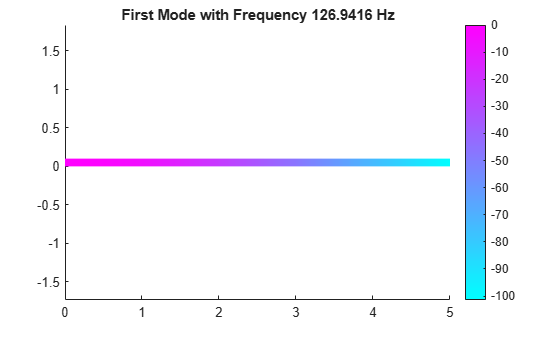Perform frequency response analysis of a tuning fork.

First, create a structural model for modal analysis of a solid tuning fork.

`model = createpde('structural','frequency-solid');`

Import the tuning fork geometry.

`importGeometry(model,'TuningFork.stl');`

Specify the Young's modulus, Poisson's ratio, and mass density to model linear elastic material behavior. Specify all physical properties in consistent units.

```structuralProperties(model,'YoungsModulus',210E9, ... 'PoissonsRatio',0.3, ... 'MassDensity',8000);```

Identify faces for applying boundary constraints and loads by plotting the geometry with the face labels.

```figure('units','normalized','outerposition',[0 0 1 1]) pdegplot(model,'FaceLabels','on') view(-50,15) title 'Geometry with Face Labels'```Impose sufficient boundary constraints to prevent rigid body motion under applied loading. Typically, you hold a tuning fork by hand or mount it on a table. To create a simple approximation of this boundary condition, fix a region near the intersection of tines and the handle (faces 21 and 22).

`structuralBC(model,'Face',[21,22],'Constraint','fixed');`

Specify the pressure loading on a tine (face 11) as a short rectangular pressure pulse. In the frequency domain, this pressure pulse is a unit load uniformly distributed across all frequencies.

```structuralBoundaryLoad(model,'Face',11,'Pressure',1); flist = linspace(0,4000,150); mesh = generateMesh(model,'Hmax',0.005); R = solve(model,2*pi*flist)```
```R = FrequencyStructuralResults with properties: Displacement: [1x1 FEStruct] Velocity: [1x1 FEStruct] Acceleration: [1x1 FEStruct] SolutionFrequencies: [1x150 double] Mesh: [1x1 FEMesh] ```

Plot the vibration frequency of the tine tip, which is face 12. Find nodes on the tip face and plot the y-component of the displacement over the frequency, using one of these nodes.

```excitedTineTipNodes = findNodes(mesh,'region','Face',12); tipDisp = R.Displacement.uy(excitedTineTipNodes(1),:); figure plot(flist,abs(tipDisp)) xlabel('Frequency'); ylabel('|Y-Displacement|');```Find the deflection of a 3-D cantilever beam under a nonuniform thermal load. Specify the thermal load on the structural model using the solution from a transient thermal analysis on the same geometry and mesh.

Transient Thermal Model Analysis

Create a transient thermal model.

`thermalmodel = createpde('thermal','transient');`

Create and plot the geometry.

```gm = multicuboid(0.5,0.1,0.05); thermalmodel.Geometry = gm; pdegplot(thermalmodel,'FaceLabels','on','FaceAlpha',0.5)```Generate a mesh.

`mesh = generateMesh(thermalmodel);`

Specify the thermal properties of the material.

```thermalProperties(thermalmodel,'ThermalConductivity',5e-3, ... 'MassDensity',2.7*10^(-6), ... 'SpecificHeat',10);```

Specify the constant temperatures applied to the left and right ends on the beam.

```thermalBC(thermalmodel,'Face',3,'Temperature',100); thermalBC(thermalmodel,'Face',5,'Temperature',0);```

Specify the heat source over the entire geometry.

`internalHeatSource(thermalmodel,10);`

Set the initial temperature.

`thermalIC(thermalmodel,0);`

Solve the model.

```tlist = [0:1e-4:2e-4]; thermalresults = solve(thermalmodel,tlist)```
```thermalresults = TransientThermalResults with properties: Temperature: [3870x3 double] SolutionTimes: [0 1.0000e-04 2.0000e-04] XGradients: [3870x3 double] YGradients: [3870x3 double] ZGradients: [3870x3 double] Mesh: [1x1 FEMesh] ```

Plot the temperature distribution for each time step.

```for n = 1:numel(thermalresults.SolutionTimes) figure pdeplot3D(thermalmodel,'ColorMapData',thermalresults.Temperature(:,n)) title(['Temperature at Time = ' num2str(tlist(n))]) caxis([0 100]) end```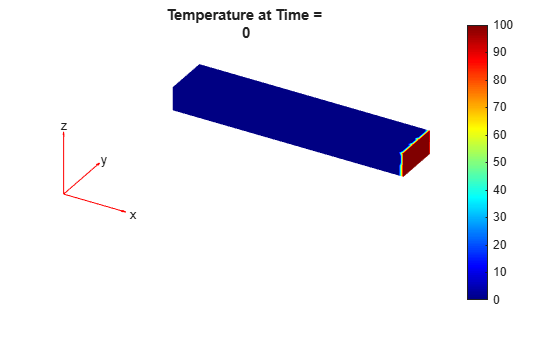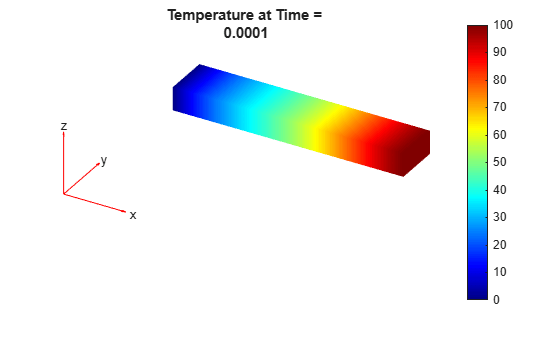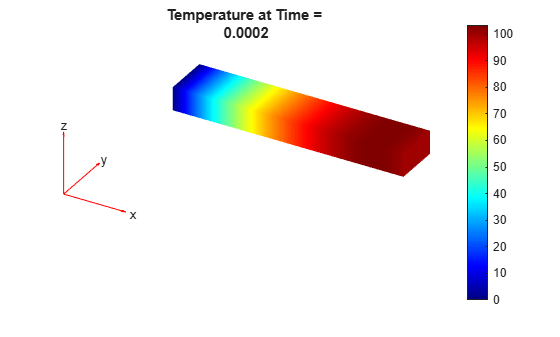Create a static structural model.

`structuralmodel = createpde('structural','static-solid');`

Include the same geometry as for the thermal model.

`structuralmodel.Geometry = gm;`

Use the same mesh that you used to obtain the thermal solution.

`structuralmodel.Mesh = mesh;`

Specify the Young's modulus, Poisson's ratio, and coefficient of thermal expansion.

```structuralProperties(structuralmodel,'YoungsModulus',1e10, ... 'PoissonsRatio',0.3, ...' 'CTE',11.7e-6);```

Apply a fixed boundary condition on face 5.

`structuralBC(structuralmodel,'Face',5,'Constraint','fixed');`

Apply a body load using the transient thermal model solution. By default, `structuralBodyLoad` uses the solution for the last time step.

`structuralBodyLoad(structuralmodel,'Temperature',thermalresults);`

Specify the reference temperature.

`structuralmodel.ReferenceTemperature = 10;`

Solve the structural model.

`thermalstressresults = solve(structuralmodel);`

Plot the deformed shape of the beam corresponding to the last step of the transient thermal model solution.

```pdeplot3D(structuralmodel,'ColorMapData',thermalstressresults.Displacement.Magnitude, ... 'Deformation',thermalstressresults.Displacement) title(['Thermal Expansion at Solution Time = ' num2str(tlist(end))]) caxis([0 3e-3])```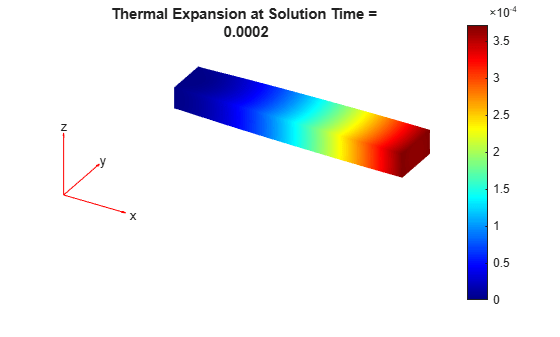Now specify the body loads as the thermal model solutions for all time steps. For each body load, solve the structural model and plot the corresponding deformed shape of the beam.

```for n = 1:numel(thermalresults.SolutionTimes) structuralBodyLoad(structuralmodel,'Temperature',thermalresults,'TimeStep',n); thermalstressresults = solve(structuralmodel); figure pdeplot3D(structuralmodel,'ColorMapData', ... thermalstressresults.Displacement.Magnitude, ... 'Deformation', ... thermalstressresults.Displacement) title(['Thermal Results at Solution Time = ' num2str(tlist(n))]) caxis([0 3e-3]) end```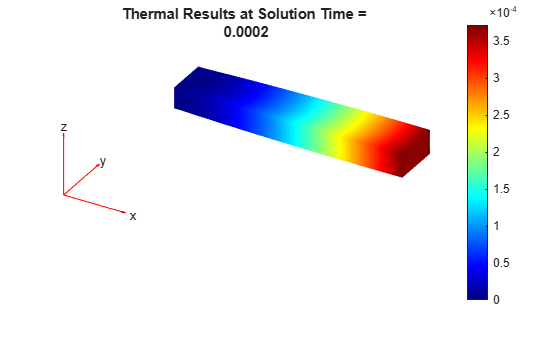Solve the for transient response at the center of a 3-D beam under a harmonic load on one of its corners.

Modal Analysis

Create a modal analysis model for a 3-D problem.

`modelM = createpde('structural','modal-solid');`

Create the geometry and include it in the model. Plot the geometry and display the edge and vertex labels.

```gm = multicuboid(0.05,0.003,0.003); modelM.Geometry = gm; pdegplot(modelM,'EdgeLabels','on','VertexLabels','on'); view([95 5])```Generate a mesh.

`msh = generateMesh(modelM);`

Specify the Young's modulus, Poisson's ratio, and mass density of the material.

```structuralProperties(modelM,'YoungsModulus',210E9, ... 'PoissonsRatio',0.3, ... 'MassDensity',7800);```

Specify minimal constraints on one end of the beam to prevent rigid body modes. For example, specify that edge 4 and vertex 7 are fixed boundaries.

```structuralBC(modelM,'Edge',4,'Constraint','fixed'); structuralBC(modelM,'Vertex',7,'Constraint','fixed');```

Solve the problem for the frequency range from 0 to 500,000. The recommended approach is to use a value that is slightly smaller than the expected lowest frequency. Thus, use `-0.1` instead of `0`.

`Rm = solve(modelM,'FrequencyRange',[-0.1,500000]);`

Transient Analysis

Create a transient analysis model for a 3-D problem.

`modelD = createpde('structural','transient-solid');`

Use the same geometry and mesh as for the modal analysis.

```modelD.Geometry = gm; modelD.Mesh = msh;```

Specify the same values for the Young's modulus, Poisson's ratio, and mass density of the material.

```structuralProperties(modelD,'YoungsModulus',210E9, ... 'PoissonsRatio',0.3, ... 'MassDensity',7800);```

Specify the same minimal constraints on one end of the beam to prevent rigid body modes.

```structuralBC(modelD,'Edge',4,'Constraint','fixed'); structuralBC(modelD,'Vertex',7,'Constraint','fixed');```

Apply a sinusoidal force on the corner opposite to the constrained edge and vertex.

`structuralBoundaryLoad(modelD,'Vertex',5,'Force',[0,0,10],'Frequency',7600);`

Specify zero initial displacement and velocity.

`structuralIC(modelD,'Velocity',[0;0;0],'Displacement',[0;0;0]);`

Specify the relative and absolute tolerances for the solver.

```modelD.SolverOptions.RelativeTolerance = 1E-5; modelD.SolverOptions.AbsoluteTolerance = 1E-9;```

Solve the model using the modal results.

```tlist = linspace(0,0.004,120); Rdm = solve(modelD,tlist,'ModalResults',Rm)```
```Rdm = TransientStructuralResults with properties: Displacement: [1x1 FEStruct] Velocity: [1x1 FEStruct] Acceleration: [1x1 FEStruct] SolutionTimes: [1x120 double] Mesh: [1x1 FEMesh] ```

Interpolate and plot the displacement at the center of the beam.

```intrpUdm = interpolateDisplacement(Rdm,0,0,0.0015); plot(Rdm.SolutionTimes,intrpUdm.uz) grid on xlabel('Time'); ylabel('Center of beam displacement')```## Input Arguments

collapse all

Static structural analysis model, specified as a `StructuralModel` object. The model contains the geometry, mesh, structural properties of the material, body loads, boundary loads, and boundary conditions.

Example: ```structuralmodel = createpde('structural','static-solid')```

Modal analysis structural model, specified as a `StructuralModel` object. The model contains the geometry, mesh, structural properties of the material, body loads, boundary loads, and boundary conditions.

Example: ```structuralmodel = createpde('structural','modal-solid')```

Transient structural analysis model, specified as a `StructuralModel` object. The model contains the geometry, mesh, structural properties of the material, body loads, boundary loads, and boundary conditions.

Example: ```structuralmodel = createpde('structural','transient-solid')```

Frequency response analysis structural model, specified as a `StructuralModel` object. The model contains the geometry, mesh, structural properties of the material, body loads, boundary loads, and boundary conditions.

Example: ```structuralmodel = createpde('structural','frequency-solid')```

Solution times, specified as a real vector of monotonically increasing or decreasing values.

Example: `0:20`

Data Types: `double`

Solution frequencies, specified as a real vector of monotonically increasing or decreasing values.

Example: `linspace(0,4000,150)`

Data Types: `double`

Frequency range, specified as a vector of two elements. Define `omega1` as slightly smaller than the lowest expected frequency and `omega2` as slightly larger than the highest expected frequency. For example, if the lowest expected frequency is zero, then use a small negative value for `omega1`.

Example: `[-0.1,1000]`

Data Types: `double`

Modal analysis results, specified as a `ModalStructuralResults` object.

Example: ```modalresults = solve(structuralmodel,'FrequencyRange',[0,1e6])```

Steady-state thermal analysis model, specified as a `ThermalModel` object. The model contains the geometry, mesh, thermal properties of the material, internal heat source, boundary conditions, and initial conditions.

Example: ```thermalmodel = createpde('thermal','steadystate')```

Transient thermal analysis model, specified as a `ThermalModel` object. The model contains the geometry, mesh, thermal properties of the material, internal heat source, boundary conditions, and initial conditions.

Example: ```thermalmodel = createpde('thermal','transient')```

## Output Arguments

collapse all

Static structural analysis results, returned as a `StaticStructuralResults` object.

Modal structural analysis results, returned as a `ModalStructuralResults` object.

Transient structural analysis results, returned as a `TransientStructuralResults` object.

Frequency response structural analysis results, returned as a `FrequencyStructuralResults` object.

Steady-state thermal analysis results, returned as a `SteadyStateThermalResults` object.

Transient thermal analysis results, returned as a `TransientThermalResults` object.

## Tips

• When you use modal analysis results to solve a transient structural dynamics model, the `modalresults` argument must be created in Partial Differential Equation Toolbox™ version R2019a or newer.

• For a frequency response model with damping, the results are complex. Use functions such as `abs` and `angle` to obtain real-valued results, such as the magnitude and phase.

#### Introduced in R2017a

##### SupportGet trial now